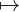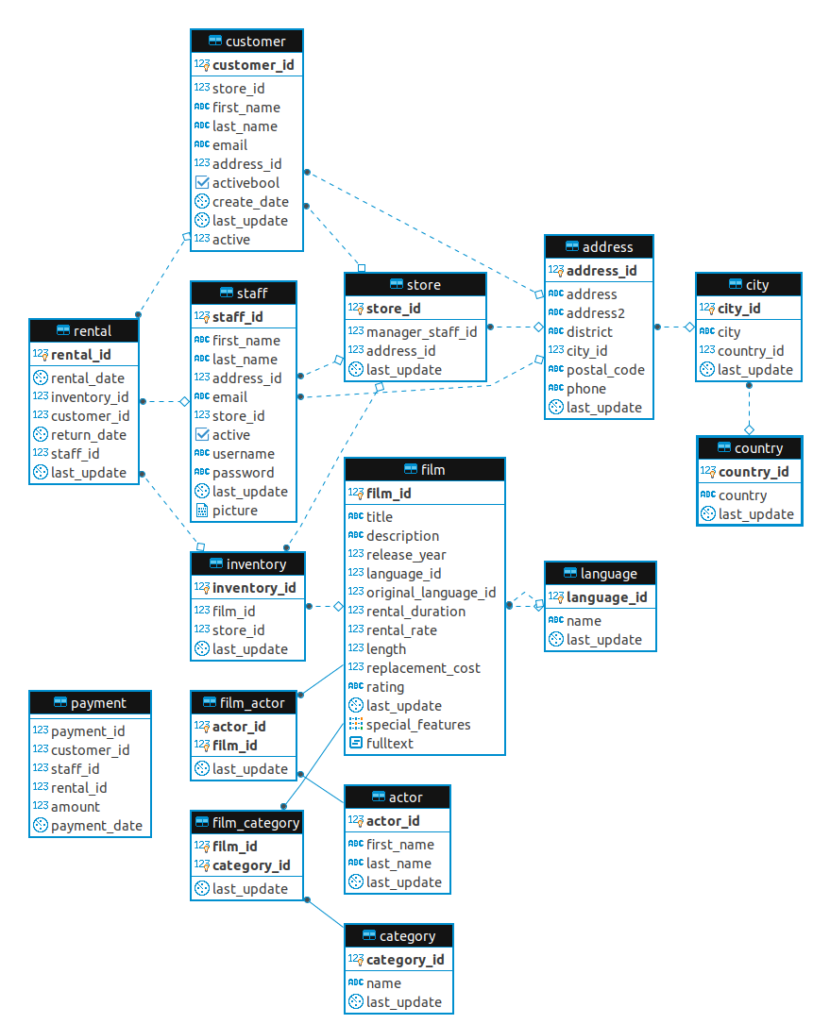# SQL – aggregate functions

Aggregate functions are very useful and popular in database queries. These functions may be general-purpose: SUM, AVG, COUNT, MAX, MIN; concatenative: ARRAY_AGG, STRING_AGG; be for statistics: CORR, STDDEV_SAMP, STDDEV_POP, VAR_SAMP, VAR_POP; be for ordered-sets: MODE, PERCENTILE_CONT; for ranking: RANK, DENSE_RANK, PERCENT_RANK, CUME_DIST; etc. There are many other aggregate functions, however, these ones seem to be the most widely-used and worth our attention at the beginning steps into Database.

There are also 2 clauses being very important for aggregation, which are GROUP BY and HAVING – to be elaborated shortly.

Table of content
General-purpose AggregationSUM and AVGCOUNTMAX and MIN
Concatenative AggregationARRAY_AGGSTRING_AGG
Aggregation for StatisticsCORRSTDDEV_SAMP, STDDEV_POP, VAR_SAMP, VAR_POP
Ordered-set AggregationMODEPERCENTILE_CONT
Hypothetical-set AggregationRANKDENSE_RANKPERCENT_RANKCUME_DIST

For illustrative purposes, we will be using the Pagila database, which is a port of the Sakila database (for MySQL) to Postgresql – the SQL version of this blog post. Pagila can be downloaded from here.Pagila’s simplified ERD

## General-purpose Aggregation

### SUM and AVG

Suppose we own a guesthouse, our service has been running for a month and generates some profits. Today, we open our computer, access our database and query the total income received. In this case, we use the SUM aggregate function. On the other hand, if we want to see how much, on average, a customer pays us for their booking, we should use the AVG function.

Example 1:

In this example, let’s calculate the total replacement cost for all films.

```SELECT SUM(replacement_cost)
FROM film;```

Note that for aggregate functions, all NULL values are excluded from the computation.

Example 2:

Let’s compute the average replacement cost, however, to make it harder, we compute the average replacement cost for each unique value of the `length` variable.

Now is when we should make use of the `GROUP BY` clause. Normally (i.e. without `GROUP BY`), the `AVG` function will calculate the average for all replacement costs in the table. Yet, with `GROUP BY col_name`, all rows with the same value for `col_name` are put into 1 group, then the average for each group is computed.

GROUP BY is placed after WHERE clause (if any) and before ORDER BY clause.

```SELECT length, AVG(replacement_cost) AS "Average replacement_cost by length"
FROM film
GROUP BY length
ORDER BY length;```

(There is a total of 140 rows, corresponding to 140 different values of `length`.)

Example 3:

However, we only want to see the replacement costs for films whose lengths are at least 90 minutes, thus we add a `HAVING` clause after the `GROUP BY`.

```SELECT length, AVG(replacement_cost) AS "Average replacement_cost by length"
FROM film
GROUP BY length
HAVING length >= 90
ORDER BY length;```

(There is a total of 96 rows.)

Note that `HAVING` acts very similar to the `WHERE` clause in terms that they both take in a boolean condition and filter the data, assuring that only the rows satisfying the condition are kept while the others are discarded. The only difference is `HAVING` is used in accompany with `GROUP BY`, i.e. WHERE is executed before grouping, while `HAVING` is executed after.

### COUNT

The `COUNT(expression)` function, as per its name, returns the count of non-null values of the `expression` inputted.

Example 1:

```SELECT COUNT(film_id)
FROM film;```

Example 2:

Let’s query the counts of address and address2 in the table `address`. We will see that the 2 counts are different, since there are some `NULL` values in address2 and `COUNT` doesn’t include NULL values.

```SELECT COUNT(address) AS count_1
, COUNT(address2) AS count_2
FROM address;```

Example 3:

When we just want to count the number of rows, the popular choices are `COUNT(1)` and `COUNT(*)`, they both provide the same result with equal performance (equal processing time).

```SELECT COUNT(1)
FROM film;```

Example 4:

Note that `COUNT` does count the duplicated values. To count only the unique values, we use `COUNT(DISTINCT expression)`.

Let’s count the number of rating categories:

```SELECT COUNT(DISTINCT rating)
FROM film;```

### MAX and MIN

Example 1:

Get the register date of the first customer (using create_date).

```SELECT MIN(create_date)
FROM customer;```

Example 2:

Over the customers who ever spent more than 10\$ for a single payment, find their lastest payment date.

```SELECT customer_id, MAX(payment_date)
FROM payment
WHERE amount > 10
GROUP BY customer_id;```

(There is a total of 107 rows.)

## Concatenative Aggregation

### ARRAY_AGG

This function collects all the values and outputs an array of those values.

Example 1:

For each customer, get the list of all inventories he/she has made a rent. Show a sample of 10 first customers.

```SELECT customer_id, ARRAY_AGG(inventory_id)
FROM rental
GROUP BY customer_id
LIMIT 10;```

Example 2:

The outputted arrays in Example 1 have their values unsorted, make them sorted.

```SELECT customer_id, ARRAY_AGG(inventory_id ORDER BY inventory_id)
FROM rental
GROUP BY customer_id
LIMIT 10;```

Example 3:

For each customer, list all staff who served him/her. Show a sample of the first 10 customers. Remember to show each staff_id at most once for each customer.

```SELECT customer_id, ARRAY_AGG(DISTINCT staff_id)
FROM rental
GROUP BY customer_id
LIMIT 10;```

### STRING_AGG

The STRING_AGG(expression, delimiter) also collects a list of values, however it then concatenate them together into a string and return this big string.

Example 1:

For each country_id, get all of its cities in the database, the cities should be listed in alphabetical order. Show a sample of the first 10 cities.

```SELECT country_id, STRING_AGG(city, ', ' ORDER BY city)
FROM city
GROUP BY country_id
LIMIT 10;```

## Aggregation for Statistics

### CORR

The `CORR(expression_1, expression_2)` function outputs the Pearson correlation coefficient of the 2 expressions over the current data (or group).

Example 1:

Calculate the correlation between film length and rental_duration to see if customers need more time to watch a longer film.

```SELECT CORR(rental_duration, length)
FROM film;```

We do have a positive correlation here, nevertheless, the value is quite small.

### VAR_SAMP

The VAR_SAMP(expression) returns the sample variance of the input values, we can use this to measure the variability of a column.

Example 1:

Get the sample variance of rental duration for each distinct film length. Show a sample of the 10 shortest lengths.

```SELECT length, VAR_SAMP(rental_duration) AS "var of rental_duration"
FROM film
GROUP BY length
ORDER BY length
LIMIT 10;```

Example 2:

Apart from VAR_SAMP, we also have VAR_POP (the population variance of input data), STDDEV_SAMP (the sample standard deviation of input data, which equals the square-root of VAR_SAMP), STDDEV_POP (the square-root of VAR_POP).

In this example, we compute the sample standard deviation of the payment amount for each customer. Show a sample of the customers with id from 50 to 60, in increasing order of id.

```SELECT customer_id, STDDEV_SAMP(amount) AS "std of amount"
FROM payment
GROUP BY customer_id
HAVING customer_id >= 50
AND customer_id <= 60
ORDER BY customer_id;```

## Ordered-set Aggregation

### MODE

`MODE() WITHIN GROUP (ORDER BY sort_expression)` returns the most frequent value amongst input values (NULL will not be selected).

Example 1:

Find the most popular length of films.

```SELECT MODE() WITHIN GROUP (ORDER BY length)
FROM film;```

### PERCENTILE_CONT

The function `PERCENTILE_CONT(fraction) WITHIN GROUP(ORDER BY sort_expression)` returns the value at the given fraction percentile of the inputs. If needed, an interpolation between adjacent values will be made.

The most frequent fractions used for this function are 0.25 (the Q1), 0.5 (the median, Q2) and 0.75 (the Q3).

Example 1:

Get the median amount of payment for each customer. Show 10 customers with the highest median pay.

```SELECT customer_id
, PERCENTILE_CONT(0.5) WITHIN GROUP (ORDER BY amount) AS "median pay"
FROM payment
GROUP BY customer_id
ORDER BY "median pay" DESC
LIMIT 10;
```

## Hypothetical-set Aggregation

### RANK

The function `RANK(args) WITHIN GROUP (ORDER BY sorted_args)` add a row containing the `args` to the table, rank the table by `sorted_args` and then output the rank of that newly added row.

Note that the rank generates gaps for duplicated rows. For example, rank [5, 9, 9, 13, 15, 15, 15, 100] gives [1, 2, 2, 4, 5, 5, 5, 8].

Example 1:

When we add a film with a length of 120 minutes to the table, then what is its rank in terms of length?

```SELECT RANK(120) WITHIN GROUP (ORDER BY length)
FROM film;```

### DENSE_RANK

This function is very similar to RANK, the difference is that it uses dense-rank – the dense rank function does not generate gaps for duplicated rows. For example, dense-rank [5, 9, 9, 13, 15, 15, 15, 100] gives [1, 2, 2, 3, 4, 4, 4, 5].

Example 1:

When we add a film with a length of 120 minutes to the table, then what is its dense-rank in terms of length?

```SELECT DENSE_RANK(120) WITHIN GROUP (ORDER BY length)
FROM film;```

### PERCENT_RANK

This function is also similar to RANK, it does exactly what RANK does, then normalizes the output value. PERCENT_RANK normalizes the output to the range [0, 1].

Example 1:

```SELECT PERCENT_RANK(120) WITHIN GROUP (ORDER BY length)
FROM film;```

### CUME_DIST

The CUME_DIST calculates the cumulative distribution. It is computed by dividing the number of rows smaller than or equal to it (including itself) for the total number of rows. Thus its output is in the range [1/n, n].

Example 1:

```SELECT CUME_DIST(120) WITHIN GROUP (ORDER BY length)
FROM film;```

## Summary

In this blog post, we introduced the common aggregate functions being used in Postgresql. Those are:

References:

• List of all aggregate functions in Postgresql: link
• Paquier’s explanation of `WITHIN GROUP`: link